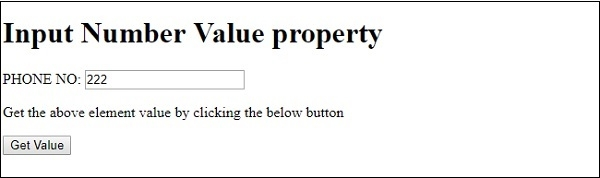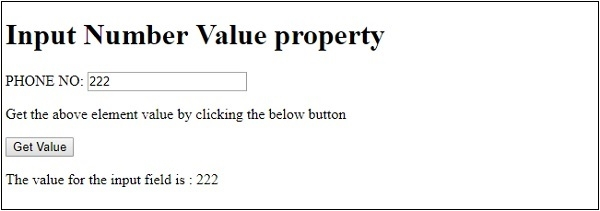# HTML DOM Input Number value Property

The HTML DOM Input number value property is associated with the input element having type=”Number” and having the value attribute. This property is used for returning the value of input element value attribute or to set it. This property is used for specifying a default value for elements and it also changes its value to user input.

## Syntax

Following is the syntax for −

Setting the value property −

numberObject.value = number;

Here, number is used for specifying the value for the number field.

## Example

Let us look at an example for the input Number value property −

Live Demo

<!DOCTYPE html>
<html>
<body>
<h1>Input Number Value property</h1>
PHONE NO: <input type="number" value="222" id="NUMBER1">
<p>Get the above element value by clicking the below button</p>
<button onclick="getValue()">Get Value</button>
<p id="Sample"></p>
<script>
function getValue() {
var t = document.getElementById("NUMBER1").value;
document.getElementById("Sample").innerHTML = "The value for the input field is : "+t;
}
</script>
</body>
</html>

## Output

This will produce the following output −On clicking the “Get Value” button −In the above example −

We have created an input field with type number and its id set to “NUMBER1”. We have specified a default value for the number field using the value attribute.

PHONE NO: <input type="number" value="222" id="NUMBER1">

We have then created the “Get Value” button that will execute the getValue() method when clicked by the user −

<button onclick="getValue()">Get Value</button>

The getValue() gets the input element using the getElementById() method and assigns its “value” attribute value to variable t. This variable is then displayed in the paragraph with id “Sample” using its innerHTML property −

function getValue() {
var t = document.getElementById("NUMBER1").value;
document.getElementById("Sample").innerHTML = "The value for the input field is : "+t;
}

Updated on: 19-Feb-2021

224 Views